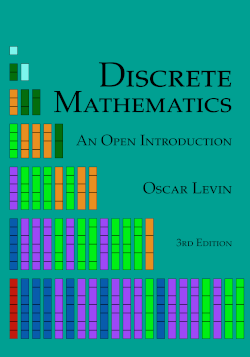# Discrete Mathematics: An Open Introduction, 3rd edition

## Chapter4Graph Theory

###### Investigate!
In the time of Euler, in the town of Königsberg in Prussia, there was a river containing two islands. The islands were connected to the banks of the river by seven bridges (as seen below). The bridges were very beautiful, and on their days off, townspeople would spend time walking over the bridges. As time passed, a question arose: was it possible to plan a walk so that you cross each bridge once and only once? Euler was able to answer this question. Are you?Graph Theory is a relatively new area of mathematics, first studied by the super famous mathematician Leonhard Euler in 1735. Since then it has blossomed in to a powerful tool used in nearly every branch of science and is currently an active area of mathematics research.
The problem above, known as the Seven Bridges of Königsberg, is the problem that originally inspired graph theory. Consider a “different” problem: Below is a drawing of four dots connected by some lines. Is it possible to trace over each line once and only once (without lifting up your pencil, starting and ending on a dot)?There is an obvious connection between these two problems. Any path in the dot and line drawing corresponds exactly to a path over the bridges of Königsberg.
Pictures like the dot and line drawing are called graphs. Graphs are made up of a collection of dots called vertices and lines connecting those dots called edges. When two vertices are connected by an edge, we say they are adjacent. The nice thing about looking at graphs instead of pictures of rivers, islands and bridges is that we now have a mathematical object to study. We have distilled the “important” parts of the bridge picture for the purposes of the problem. It does not matter how big the islands are, what the bridges are made out of, if the river contains alligators, etc. All that matters is which land masses are connected to which other land masses, and how many times.
We will return to the question of finding paths through graphs later. But first, here are a few other situations you can represent with graphs:

## Example4.0.1.

Al, Bob, Cam, Dan, and Euler are all members of the social networking website Facebook. The site allows members to be “friends” with each other. It turns out that Al and Cam are friends, as are Bob and Dan. Euler is friends with everyone. Represent this situation with a graph.
Solution.
Each person will be represented by a vertex and each friendship will be represented by an edge. That is, two vertices will be adjacent (there will be an edge between them) if and only if the people represented by those vertices are friends.## Example4.0.2.

Each of three houses must be connected to each of three utilities. Is it possible to do this without any of the utility lines crossing?
Solution.
We will answer this question later. For now, notice how we would ask this question in the context of graph theory. We are really asking whether it is possible to redraw the graph below without any edges crossing (except at vertices). Think of the top row as the houses, bottom row as the utilities.